# Experiment_730_Molecular Structure and Isomerism 1_2_1

$$\newcommand{\vecs}{\overset { \rightharpoonup} {\mathbf{#1}} }$$ $$\newcommand{\vecd}{\overset{-\!-\!\rightharpoonup}{\vphantom{a}\smash {#1}}}$$$$\newcommand{\id}{\mathrm{id}}$$ $$\newcommand{\Span}{\mathrm{span}}$$ $$\newcommand{\kernel}{\mathrm{null}\,}$$ $$\newcommand{\range}{\mathrm{range}\,}$$ $$\newcommand{\RealPart}{\mathrm{Re}}$$ $$\newcommand{\ImaginaryPart}{\mathrm{Im}}$$ $$\newcommand{\Argument}{\mathrm{Arg}}$$ $$\newcommand{\norm}{\| #1 \|}$$ $$\newcommand{\inner}{\langle #1, #2 \rangle}$$ $$\newcommand{\Span}{\mathrm{span}}$$ $$\newcommand{\id}{\mathrm{id}}$$ $$\newcommand{\Span}{\mathrm{span}}$$ $$\newcommand{\kernel}{\mathrm{null}\,}$$ $$\newcommand{\range}{\mathrm{range}\,}$$ $$\newcommand{\RealPart}{\mathrm{Re}}$$ $$\newcommand{\ImaginaryPart}{\mathrm{Im}}$$ $$\newcommand{\Argument}{\mathrm{Arg}}$$ $$\newcommand{\norm}{\| #1 \|}$$ $$\newcommand{\inner}{\langle #1, #2 \rangle}$$ $$\newcommand{\Span}{\mathrm{span}}$$$$\newcommand{\AA}{\unicode[.8,0]{x212B}}$$

 Student Name Laboratory Date:  Date Report Submitted: ___________________________ Student ID Experiment Number and Title Experiment 730:  Molecular Structure and Isomerism

Experiment 730:  Molecular Structure and Isomerism

Section 1:  Purpose and Summary

• Construct models of alkanes, haloalkanes, alkenes, simple amino acids and isomeric compounds.

• Use a molecular model kit to construct these molecules and visualize their structure and three-dimensional shape.

• Write structural formulas and name alkanes, haloalkanes, cis- and trans- isomers and optically active compounds.

Alkanes are saturated hydrocarbons. They contain only carbon and hydrogen and have no multiple (double or triple) bonds present. Cycloalkanes are alkanes containing carbon atoms connected in an enclosed (ring) structure. Haloalkanes are molecules that contain carbon, hydrogen, and one or more halogen atoms (F, Cl, Br, or I) as substituents.

Recall that when drawing Lewis structures, a carbon atom will always form four bonds, a hydrogen atom will form one bond and can never be a “central atom”, and a halogen atom will form one bond and have three lone pairs of electrons.

To visualize the three-dimensional structures of organic compounds, it is helpful to build models of the molecules using a molecular model kit. Ball-and-stick model kits contain wooden or plastic balls representing atoms, with a different color for each element, and wooden or plastic sticks that represent bonds. Springs or longer flexible sticks may be used to represent double or triple bonds.

There are various types of formulas that can be written for a particular molecule. The complete structural formula shows all the atoms and how they are exactly connected. However, it is somewhat abbreviated and does not give you much three-dimensional information. Atoms are grouped and every bond is not explicitly shown, but the connections between atoms are implied based on how it is written. The zigzag or line-angle formula is even more abbreviated: carbon atoms are assumed to be at the ends and at each bend or angle in the structure, and hydrogen atoms are not shown, but implied. The assumption is that each carbon makes four bonds, so if one bond is shown for a particular carbon atom, it is implied that three hydrogen atoms are also bonded to that carbon (to make a total of four bonds). On the other hand, the molecular formula does not give any structural information. It just specifies the total number of each type of atom present in the compound. Shown below are various formulas for propane.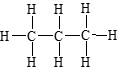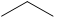CH3CH2CH3         C3H8

complete structural          condensed structural          zigzag or line-angle          Molecular formula

formula                          formula

The names and formulas of some common alkanes are shown in the table below. See your textbook or lecture notes for the IUPAC guidelines in naming organic compounds.

 Name Condensed Structural Formula Methane CH4 Ethane CH3CH3 Propane CH3CH2CH3 Butane CH3CH2CH2CH3 Pentane CH3CH2CH2CH2CH3 Hexane CH3CH2CH2CH2CH2CH3 Heptane CH3CH2CH2CH2CH2CH2CH3 Octane CH3CH2CH2CH2CH2CH2CH2CH3 Nonane CH3CH2CH2CH2CH2CH2CH2CH2CH3 Decane CH3CH2CH2CH2CH2CH2CH2CH2CH2CH3

For most organic compounds, if the atoms are arranged differently, a different compound results. Molecules which have the same molecular formulas, but different connectivity of the atoms are called structural isomers of each other. Isomers exhibit different properties (such as melting point and boiling point) and have different names.

For example, for butane, (C4H10), there are two possible ways of arranging the atoms. The first is to have all four carbon atoms connected in an unbroken line. This is called “n-butane”. The second is to have three carbons connected in a sequence with the fourth carbon attached to the middle carbon. The common name for this molecule is “isobutane”. The structures of n-butane and isobutane are shown below. Note that since the two molecules have the same molecular formula but different structures, they are considered isomers of each other.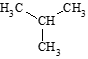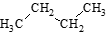n-butane isobutane

Another type of isomerism observed in organic compounds is stereoisomerism. Stereoisomers have the same molecular formulas and same connectivity of the atoms, but different spatial arrangement of the atoms. Geometric isomers, a type of stereoisomers, show two substituents in the same side (cis) or opposite sides (trans) of a carbon-carbon double bond or a ring structure. For example, in 2-butene, CH3CH=CHCH3, the cis isomer has the two methyl groups on the same side of the double bond, while the trans isomer has the two methyl groups on the opposite sides of the double bond.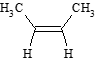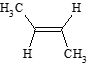cis-2-butene    trans-2-butene

Another type of stereoisomers are optical isomers where substituents on a tetrahedral carbon (stereocenter) are arranged in such a manner that the mirror images of the molecular structure are non-superimposable. They are also called enantiomers. Optical isomers are common in biological systems.  There is a standard naming convention for them. The R (‘right-handed’) configuration and the S (‘left-handed’) configuration which depend on the priority rules on the groups bonded to the stereocenter.

Section 2: Procedure

Use your molecular model kit to construct a three-dimensional model of each molecule in this experiment.

The following is a quick guide to using your molecular model kit:

• Each colored center corresponds to a specific atom.

• Refer to the color key provided in the kit, but generally the color scheme is as follows:

1. Black is for Carbon

1. White is for Hydrogen

1. Red is for Oxygen

1. Blue is for Nitrogen

1. Green/Orange/Purple is for a halogen

1. Yellow is for sulfur

• Use the medium length gray links for single bonds.

• Use the short white links for lone pairs.

• Use the flexible long gray links for making double or triple bonds.

Part 1: Alkanes

 Construct a model and sketch a 3-D representation for methane (CH4). Complete the worksheet below (starting in Section 3) by writing the complete structural formula and the condensed structural formula for methane. Repeat step 2 for ethane and propane. Notice that all the bond angles are equivalent for each molecule. From the Valence Shell Electron Pair Repulsion (VSEPR) model, the bond angle for all carbon atoms is predicted to be 109.5o and the geometry is tetrahedral. Repeat step 2 for butane. Notice that in the 3-D structure, the carbons are not actually arranged in a straight line. Using your model for butane, rotate the bonds and twist the molecule. There is free rotation around single bonds, so molecules are constantly twisting, rotating, and changing their conformation. It is important to understand that this type of motion is happening constantly in any given molecule. By looking at the following structures, you should be able to recognize that they are equivalent and represent the same molecule. They are NOT isomers of each other!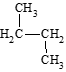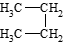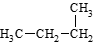Construct a model for isobutane. (The IUPAC name for isobutane is 2-methylpropane.) Compare this with your model for butane from step 5. Note that butane and isobutane cannot be interconverted without breaking the bonds. Thus, they represent distinct molecules with different properties. Draw the complete structural formula and the condensed structural formula for isobutane in the worksheet below. Locate the chemistry handbook in the lab. Look up the molar mass, melting point, boiling point, and density for butane and for isobutane (which might be listed as 2-methylpropane). Record the data in the worksheet below. Now, consider pentane, C5H12. There are three isomers for pentane. Construct a model for each isomer. For each isomer, write the condensed structural formula and IUPAC name. Look up the molar mass, melting point, boiling point, and density for each isomer and record below.

Part 2Cycloalkanes

Construct models of cyclopropane, cyclobutane, and cyclopentane. You will need to use flexible sticks for the carbon-carbon bonds in the first two molecules because their bond angles are significantly different from the normal 109.5°. Cyclopropane and cyclobutane have a significant amount of “ring strain.” Draw the complete structural formula, the condensed structural formula, and the line-angle formula for each. Record your data in the worksheet below.

Part 3: Haloalkanes

 Starting with a model of methane (CH4), replace one hydrogen atom with a chlorine (green) atom. You have made a model of CH3Cl (chloromethane). Does it matter which hydrogen is replaced? All hydrogen atoms in CH4 are considered equivalent. Each one has the same angle and position relative to the other hydrogen atoms in the molecule. Each one is equidistant from all the others. Again, starting with a model of CH4, replace two hydrogen atoms with chlorine atoms. Are there different isomers possible for this formula? Given the structures below, are these molecules isomers or equivalent? Explain.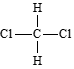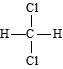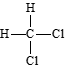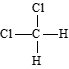Go back to your model of ethane, CH3CH3. Are all the hydrogen atoms equivalent? Explain. Starting with your model of ethane, make a model of chloroethane, CH3CH2Cl. Again, do this by removing one of the hydrogen atoms and replacing it with a chlorine atom. Are there different isomers possible for this molecule? Explain. Draw the three-dimensional structure of this molecule. From your model in step 4, replace another hydrogen atom with a chlorine atom. There are two possible locations for the second chlorine atom: on the same carbon as the first chlorine atom, or on the other carbon atom. Thus, there are two isomers possible for the molecular formula C2H4Cl2. Make models of both isomers and compare them.    Notes: There are only two isomers possible for this molecule. There are five possible locations for the second chlorine atom. But since two of the positions are equivalent to each other (free rotation around the bonds), placing the chlorine at either of these two positions results in the same molecule. Similarly, the other three positions are equivalent to each other, so placing the chlorine at any of these positions will give you the same molecule. Convince yourself of this by making various models of the different possibilities and noticing which ones are the same. Even though there are five possible locations for the chlorine, there are only two types of positions, hence, only two isomers are possible. Draw the condensed structural and line-angle formulas for the two isomers and give their IUPAC names in the worksheet below. How many isomers are possible for the molecular formula C3H6Cl2? Draw and name each isomer in the worksheet below. Make models of the molecules. Make sure that all the isomers you list are different molecules.

Part 4Geometric isomerism

 Starting with a model of ethane CH3CH3.  Remove two of the hydrogens.  Replace the hydrogens with a second bond between the two carbons using one of the longer bonds in the model kit.  This new compound is called ethylene.   It is sometimes written as H2C=CH2.  It has a carbon-carbon double-bond.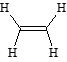Remove a hydrogen and replace it with a chlorine.  This formula is CH2CHCl and is called chloroethylene. The double bond restricts where the chlorine can be and since the molecule cannot be turned around in any geometric position, there is only one way that chloroethylene can be constructed. There are 3 hydrogens remaining on chloroethylene.  Remove the hydrogen from the carbon that already has one chlorine attached to it and replace it with chlorine.  This molecule is called 1,1-dichloroethylene and can also be written CH2CCl2.   There is only one way to construct this molecule, and the 1’s indicate that the two atoms of chlorine are bonded to the same carbon. Swap one of your chlorines with a hydrogen from the second carbon in 1,1-dichloroethylene.  There are two possible ways to construct this molecule.  Make models of the isomers and compare them. Which one is cis and which one is trans? Construct models of the cis and trans isomers of 2-pentene. See how these two compounds are geometrically bonded differently. They have slightly different chemical properties and chemical reactivity. Draw their structures in the worksheet below.

Part 5Optically active centers

 Starting with a model of CH4, replace the 1st  hydrogen atom with a chlorine atom, a 2nd hydrogen with a bromine atom, and a 3rd hydrogen with an iodine atom.  The formula for this is CHClBrI.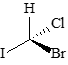Construct a second model of CHClBrI that is identical to the first one.  You should be able to see that the two models can be superimposed on one another. With one of your models of CHClBrI, swap any two of the atoms.  For example, the H and Cl can be exchanged with one another but do not change the Br and I. Compare your two models of CHClBrI.  Note that these compounds are now different.  It is impossible to superimpose one upon the other.  They are, in effect, mirror images of each other relative to the central carbon atom.      Notes: There are only two isomers possible for this molecule. Convince yourself of this by making various models of the different possibilities and noticing which ones are the same. Even though there are four different atoms attached to the central carbon, only two isomers are possible. Look down the bond that has the highest priority.  In CHClBrI, this would be the carbon-iodine bond (carbon is bonded to the element with the highest atomic number). Assign priority to the remaining 3 bonds.  In this case, the order would be C-Br > C-Cl >C-H.  Draw an arrow in a circle around the 3 bonds.  If the arrow is clockwise, it is an R- configuration.  If it is counterclockwise, it is an S- configuration.  Assign the R- and S- configuration to your two isomers.

Part 6Amino acids.

 Nitrogen bonds similarly to carbon, but it has an additional lone pair of electrons.  This lone pair behaves just like a bonding atom—it occupies space at this tetrahedron.  Start with a methane, CH4.  Remove a hydrogen and replace it with a nitrogen, N.  Add two more hydrogens on the nitrogen.  You now have made a model of nitromethane, CH3NH2.  Notice how the lone pair of electrons on the nitrogen occupies a bonding site and affects the geometry of the molecule.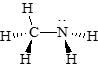Oxygen had two lone pairs of electrons.  Make acetic acid, CH3COOH.  In this case, one of the oxygens is double-bonded to a carbon (like the double-bond formed in part 4, but with two different atoms.  The other oxygen is single-bonded to the carbon and has a terminal hydrogen.

 Amino acids have a general formula of H2NCHRCOOH, where R may be different for different amino acids.  The simplest amino acid is glycine, H2NCH2COOH.   Using your model kit, build glycine.    Note that glycine does not have an optically active center (there are no carbon centers with four different things bonded to it). Now remove one of the hydrogens off the CH2- group in glycine and replace it with a CH3- group.  The new amino acid is called alanine, H2NCHCH3COOH.  It has an optically active center.  Determine if this is an R- or S- version that your model represents. Remove one of the hydrogens from the methyl group you just added and replace it with a benzene ring (C6H5-).  This amino acid is called phenylalanine, H2NCH(CH2C6H5)COOH.  Assign the R- and S- configuration to your structure.

Section 3: Worksheet

Part 1: Alkanes

1. CH4

 3D sketch: Complete structural formula:        Condensed structural formula:

1. C2H6

 3D sketch: Complete structural formula:        Condensed structural formula:

1. C3H8

 3D sketch: Complete structural formula:        Condensed structural formula:

1. butane (C4H10)

 3D sketch: Complete structural formula:        Condensed structural formula: Molar mass:                     Melting point:                     Boiling point:                      Density:
1. isobutane (C4H10)

 3D sketch: Complete structural formula:        Condensed structural formula: Molar mass:                     Melting point:                     Boiling point:                      Density:

1. Isomers of pentane, C5H12

 Condensed structural formula IUPAC name Molar mass Melting point Boiling point Density Isomer #1 Isomer #2 Isomer #3

Part 2: Cycloalkanes

 Complete structural formula Condensed structural formula Line-angle formula cyclopropane cyclobutene cyclopentane

Part 3: Haloalkanes

1. Isomers of C2H4Cl2

 IUPAC Name Condensed structural formula Line-angle formula Isomer #1 Isomer #2

1. Isomers of C3H6Cl2

Part 4: Geometric Isomers

Isomers of 2-pentene

 IUPAC Name Structural or line-angle formula Isomer #1 Isomer #2

Part 5: Optically active isomers

Isomers of CHClBrI  (Draw bow-tie structures.)

 Isomer #1 Isomer #2

Part 6: Amino Acids

Draw bow-tie structures for each compound.

 Alanine Phenylalanine

Post Lab Questions:

1. For each of the following pairs of molecules, state whether they are isomers, identical, or neither. Briefly explain your reasoning in each case.

1.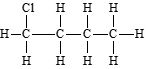and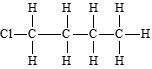1.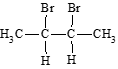and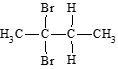1.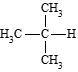and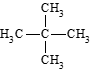1.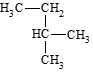and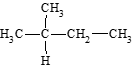1.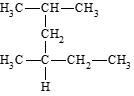and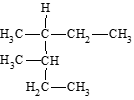1.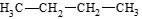and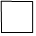1. Name each of the molecules in question #1 above.

1. Name each of the following molecules.

1.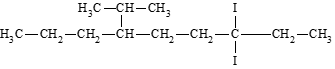1.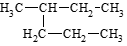1.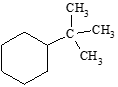1. Draw condensed structural formulas for:

1. 3-ethylpentane                                                    c) 1,2-dibromobutane

1. 1-bromo-3-chlorocyclopentane                          d) methylcyclohexane

1. Write the condensed structural formulas for all isomers of C4H9Br. Name each isomer.

1. Write the condensed structural formulas for the cis and trans isomers of 1-chloro-2-methyl-2-butene.

1. Draw the enantiomers of 1-bromo-2-chloropropane. Identify the R- and S- isomers.

Notes:

Experiment_730_Molecular Structure and Isomerism 1_2_1 is shared under a CC BY license and was authored, remixed, and/or curated by LibreTexts.